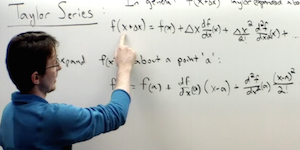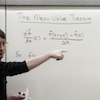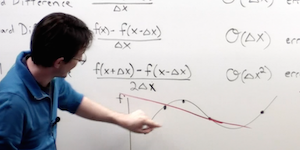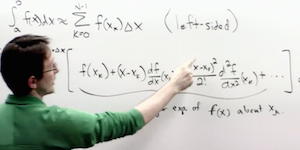# Beginning Scientific Computing

## Integration and Differentiation

### Lecture 1### [ view ]

NUMERICAL DIFFERENTIATION METHODS: From simple Taylor series expansions, the theory of numerical differentiation is developed.

MATLAB COMMANDS

`n/a`

MATLAB CODE

### Supplementary VideosView
Mean Value Theorem

### Lecture 2### [ view ]

HIGHER-ORDER ACCURACY SCHEMES FOR DIFFERENTIATION AND INTEGRATION: The accuracy of the differentiation approximations is considered and new schemes are developed to lower the error. Integration is also introduced as a numerical algorithm.

MATLAB COMMANDS

`n/a`

MATLAB CODE

### Lecture 3### [ view ]

HIGHER-ORDER INTEGRATION SCHEMES: Higher-order numerical integration schemes are considered along the classic schemes of trapezoidal rule and Simpson’s rule.

MATLAB COMMANDS

`TRAPZ CUMTRAPZ QUAD`

MATLAB CODE

© 2015 kutz